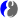# Tutorial:RTextDoc/LaTeX

You can work with LaTeX documents and convert them to HTML/XML pages. This functionality does not require installedLaTeX. Such conversion has its limitations which are described in Snuggletex web page.

Here is a simple example taken directly from Snuggletex web page.

<file latex example.tex> \section*{Welcome to SnuggleTeX!}

\begin{itemize}

 \item This is an itemized list.

 \item This is some inline maths: $x^2+ y^2 = 1$

 \item Some block maths:
$\sum_{n=1}^\infty \frac{1}{n^2} = \frac{\pi^2}{6}$
(\textbf{Note}: This currently doesn't display nicely in Firefox 3. Boo\ldots)

 \item A $2\times 2$ matrix created via a user-defined command:
\newcommand{\mat}{\left( \begin{array}{cc} #1 & #2 #3 & #4 \end{array} \right)}
$A = \mat{\alpha}{\beta}{\gamma}{\delta}$


\end{itemize} </file>

Here is an example of a SnuggleTeX error message. You can set it up to display the messages inline (as we have here) or report them in various other ways: </jcode>

Create a file "test.tex" with these lines, open in RTextDoc and convert to HTML (the menu "Convert→LaTeX to HTML"). You will see a new HTML file in the editor, which is also opened in a Browser.